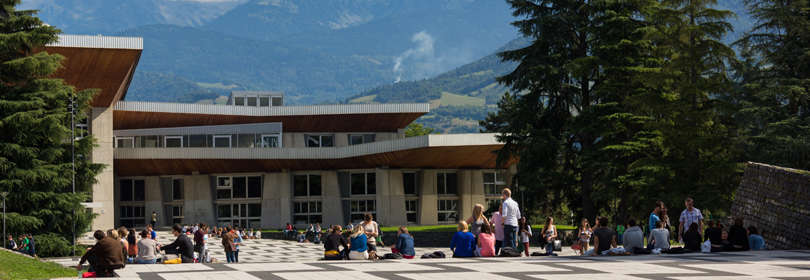Our engineering & Master degrees

School of engineering in Physics, Applied Physics, Electronics & Materials
Science# Modélisation paramétrique - NL - 5PMSMNL0

A+Augmenter la taille du texteA-Réduire la taille du texteImprimer le documentEnvoyer cette page par mail cet article Facebook Twitter Linked In
• #### Number of hours

• Lectures : 16.0
• Tutorials : 4.0
ECTS : 1.5

### Goals

The aim of this lecture is to provide basic elements of dynamical system modeling in both discrete and continuous time system analysis framework.

In a second part of the lecture, non linear optimal filtering problems are studied in a Bayesian context. Classical formulation of Kalman optimal filtering are obtained in this framework, and generalization are discussed. Some elements on particle filtering approaches are given.

Contact Olivier MICHEL

Content

A. Discrete time (DT) random processes and dynamical systems

1. DT dynamical system and filters : definitions
2. Spectral factorization and second order properties
3. Dyn. System models for DT random processes, linear case
1. Wold theorem, AR, ARMA models.
2. State space representation of random processes
4. DT Markov processes

B. Continuous time (CT) dynamical systems, general case

1. Motivations, Langevin equation
2. Random walks, Brownian motion
3. Mean square calculations for 2nd order processes
4. Applications to CT dyn. syst. modeling
5. notes on Itô integral.
6. Itô stochastic calculations
7. Fokker-Planck equation

C. Optimal filtering

1. Definitions
2. Probabilistic approach, def. of optimality

D. Non linear optimal filtering

1. Continuous-Discrete time approach
1. Model
2. Conditional pdfs
3. Moments
2. Discrete-Discrete filter case

E. Linear filtering as a particular case : Kalman-Bucy

F. Particle filters

Prerequisites

Tests